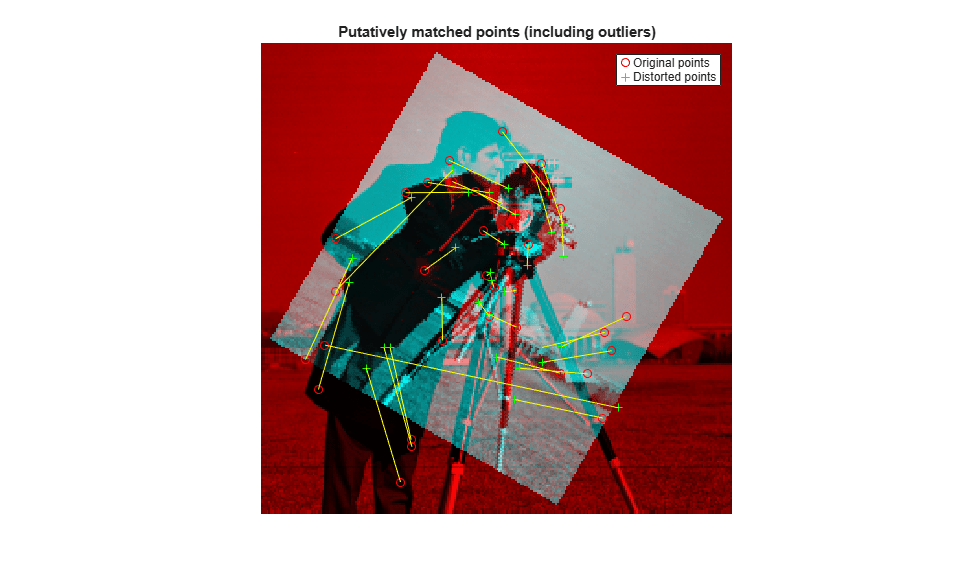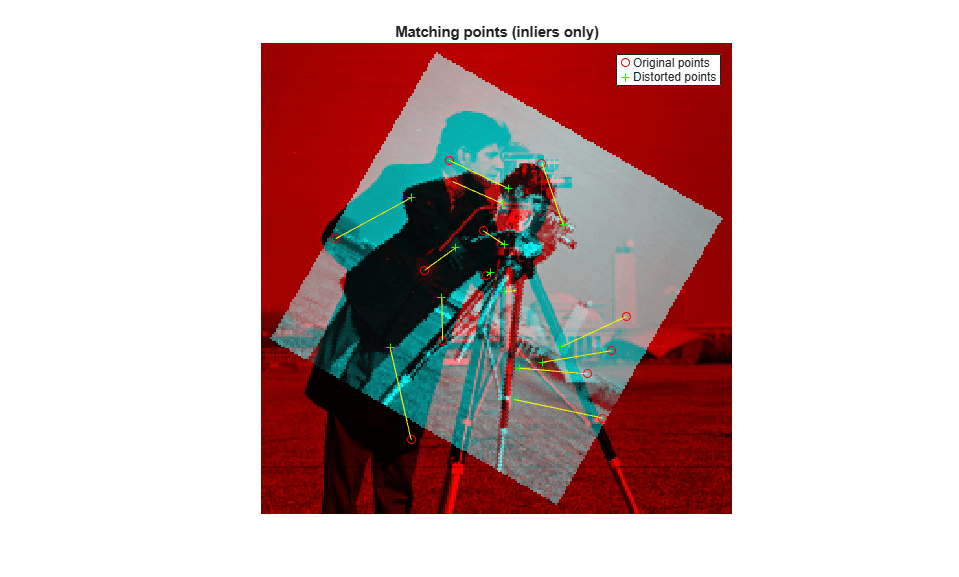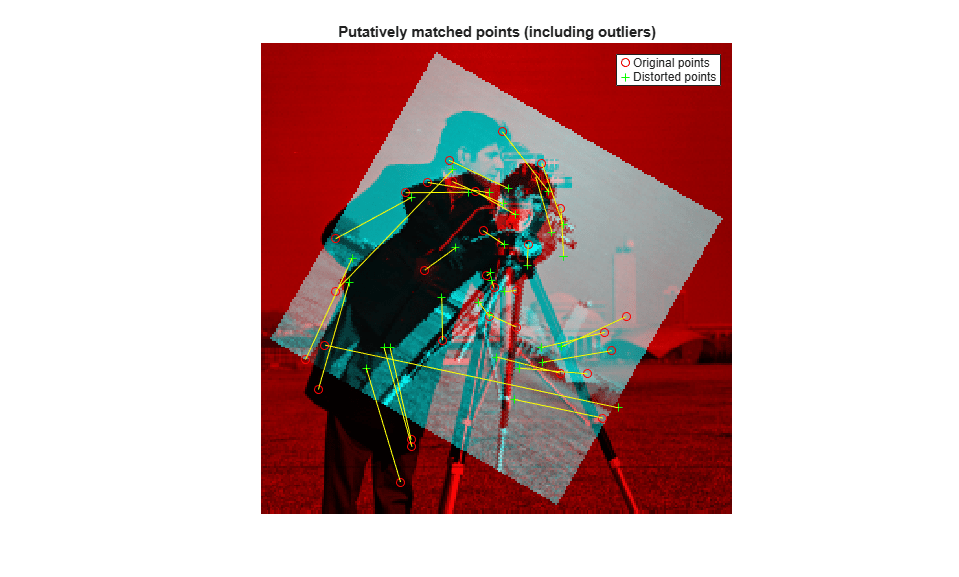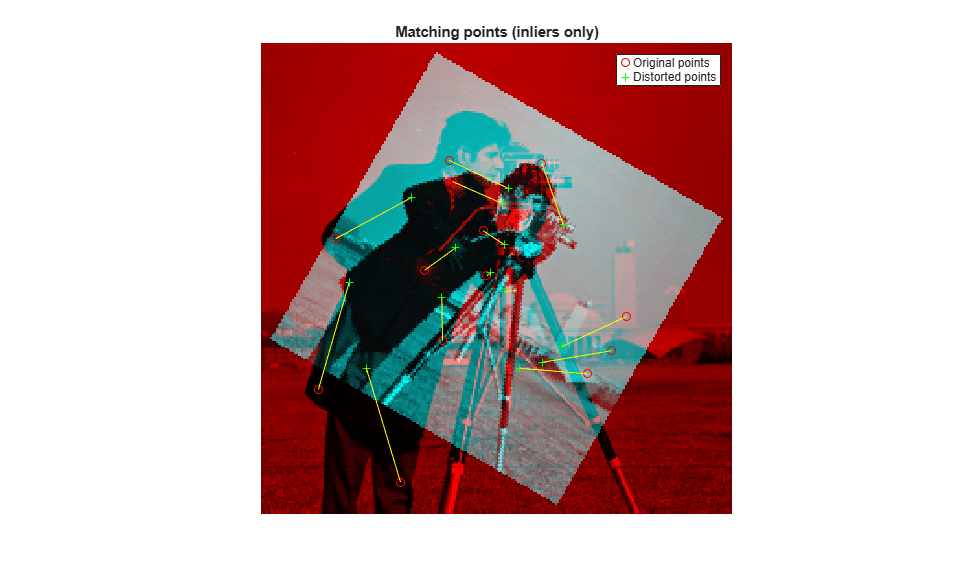Documentation

## Introduction to Code Generation with Feature Matching and Registration

This example shows how to use the MATLAB® Coder™ to generate C code for a MATLAB file. The example explains how to modify the MATLAB code used by the Automated Feature Matching example so that it is supported for code generation. The example highlights some of the general requirements for code generation, as well as some of the specific actions you must take to prepare MATLAB code. Once the MATLAB code is ready for code generation, you use the `codegen` command to generate a C-MEX function. Finally, to verify results, the example shows you how to run the generated C-MEX function in MATLAB and compare its output with the output of the MATLAB code.

This example requires a MATLAB Coder license.

### Set Up Your C Compiler

To run this example, you must have access to a C compiler and you must configure it using 'mex -setup' command. For more information, see Setting Up Your C Compiler.

### Decide Whether to Run Under MATLAB or as a Standalone Application

Generated code can run inside the MATLAB environment as a C-MEX file, or outside the MATLAB environment as a standalone executable or shared utility to be linked with another standalone executable. For more details about setting code generation options, see the -config option of the `codegen` command.

MEX Executables

This example generates a MEX executable to be run inside the MATLAB environment.

Generating a C-MEX executable to run inside of MATLAB can also be a great first step in a workflow that ultimately leads to standalone code. The inputs and the outputs of the MEX-file are available for inspection in the MATLAB environment, where visualization and other kinds of tools for verification and analysis are readily available. You also have the choice of running individual commands either as generated C code, or via the MATLAB engine. To run via MATLAB, declare relevant commands as `extrinsic`, which means that the generated code will re-enter the MATLAB environment when it needs to run that particular command. This is useful in cases where either an isolated command does not yet have code generation support, or if you wish to embed certain commands that do not generate code (such as plot command).

Standalone Executables

If deployment of code to another application is the goal, then a standalone executable will be required. The first step is to configure MATLAB Coder appropriately. For example, one way to tell it you want a standalone executable is to create a MATLAB Coder project using the MATLAB Coder IDE and configure that project to generate a module or an executable. You can do so using the C/C++ static library or C/C++ executable options from the Build type widget on the Generate page. This IDE is available by navigating as follows:

- Click APPS tab - Scroll down to MATLAB Coder - In MATLAB Coder Project dialog box, click OK

You can also define a config object using

`a=coder.config('exe')`

and pass that object to the coder command on the MATLAB command line. When you create a standalone executable, you have to write your own main.c (or main.cpp). Note that when you create a standalone executable, there are no ready-made utilities for importing or exporting data between the executable and the MATLAB environment. One of the options is to use printf/fprintf to a file (in your handwritten main.c) and then import data into MATLAB using 'load -ascii' with your file.

### Break Out the Computational Part of the Algorithm into a Separate MATLAB Function

MATLAB Coder requires MATLAB code to be in the form of a function in order to generate C code. Note that it is generally not necessary to generate C code for all of the MATLAB code in question. It is often desirable to separate the code into the primary computational portion, from which C code generation is desired, and a harness or driver, which does not need to generate C code - that code will run in MATLAB. The harness may contain visualization and other verification aids that are not actually part of the system under test. The code for the main algorithm of this example resides in a function called visionRecovertformCodeGeneration_kernel.m

Once the code has been re-architected as described above, you must check that the rest of the code uses capabilities that are supported by MATLAB coder. For a list of supported commands, see MATLAB Coder documentation. For a list of supported language constructs, see MATLAB Language Features Supported for C/C++ Code Generation.

It may be convenient to have limited visualization or some other capability that is not supported by the MATLAB Coder present in the function containing the main algorithm, which we hope to compile. In these cases, you can declare these items 'extrinsic' (using coder.extrinsic). Such capability is only possible when you generate the C code into a MATLAB MEX-file, and those functions will actually run in interpreted MATLAB mode. If generating code for standalone use, extrinsic functions are either ignored or they generate an error, depending on whether the code generation engine determines that they affect the results. Thus the code must be properly architected so that the extrinsic functions do not materially affect the code in question if a standalone executable is ultimately desired.

The original example uses showMatchedFeatures and imshowpair routines for visualization of the results. These routines are extracted to a new function featureMatchingVisualization_extrinsic.m. This function is declared extrinsic.

### Run the Simulation

The kernel file visionRecovertformCodeGeneration_kernel.m has two input parameters. The first input is the original image and the second input is the image distorted by rotation and scale.

```% define original image original = imread('cameraman.tif'); % define distorted image by resizing and then rotating original image scale = 0.7; J = imresize(original, scale); theta = 30; distorted = imrotate(J, theta); % call the generated mex file [matchedOriginalLoc, matchedDistortedLoc,... thetaRecovered, ... scaleRecovered, recovered] = ... visionRecovertformCodeGeneration_kernel(original, distorted); ```
```scaleRecovered = 0.701003 thetaRecovered = 30.235065 ```### Compile the MATLAB Function Into a MEX File

Now use the codegen function to compile the visionRecovertformCodeGeneration_kernel function into a MEX-file. You can specify the '-report' option to generate a compilation report that shows the original MATLAB code and the associated files that were created during C code generation. You may want to create a temporary directory where MATLAB Coder can create new files. Note that the generated MEX-file has the same name as the original MATLAB file with _mex appended, unless you use the -o option to specify the name of the executable.

MATLAB Coder requires that you specify the properties of all the input parameters. One easy way to do this is to define the input properties by example at the command-line using the -args option. For more information see Input Specification. Since the inputs to visionRecovertformCodeGeneration_kernel.m are a pair of images, we define both the inputs with the following properties:

• variable-sized at run-time with upper-bound [1000 1000]

• data type uint8

```% Define the properties of input images imageTypeAndSize = coder.typeof(uint8(0), [1000 1000],[true true]); compileTimeInputs = {imageTypeAndSize, imageTypeAndSize}; codegen visionRecovertformCodeGeneration_kernel.m -report -args compileTimeInputs; ```
```Code generation successful: To view the report, open('codegen/mex/visionRecovertformCodeGeneration_kernel/html/report.mldatx'). ```

### Run the Generated Code

```[matchedOriginalLocCG, matchedDistortedLocCG,... thetaRecoveredCG, scaleRecoveredCG, recoveredCG] = ... visionRecovertformCodeGeneration_kernel_mex(original, distorted); ```
```scaleRecovered = 0.701003 thetaRecovered = 30.235065 ```### Clean Up

```clear visionRecovertformCodeGeneration_kernel_mex; ```

### Compare Codegen with MATLAB Code

Recovered scale and theta for both MATLAB and CODEGEN, as shown above, are within reasonable tolerance. Furthermore, the matched points are identical, as shown below:

```isequal(matchedOriginalLocCG, matchedOriginalLoc) isequal(matchedDistortedLocCG, matchedDistortedLoc) ```
```ans = logical 1 ans = logical 1 ```

### Appendix

The following helper functions are used in this example.